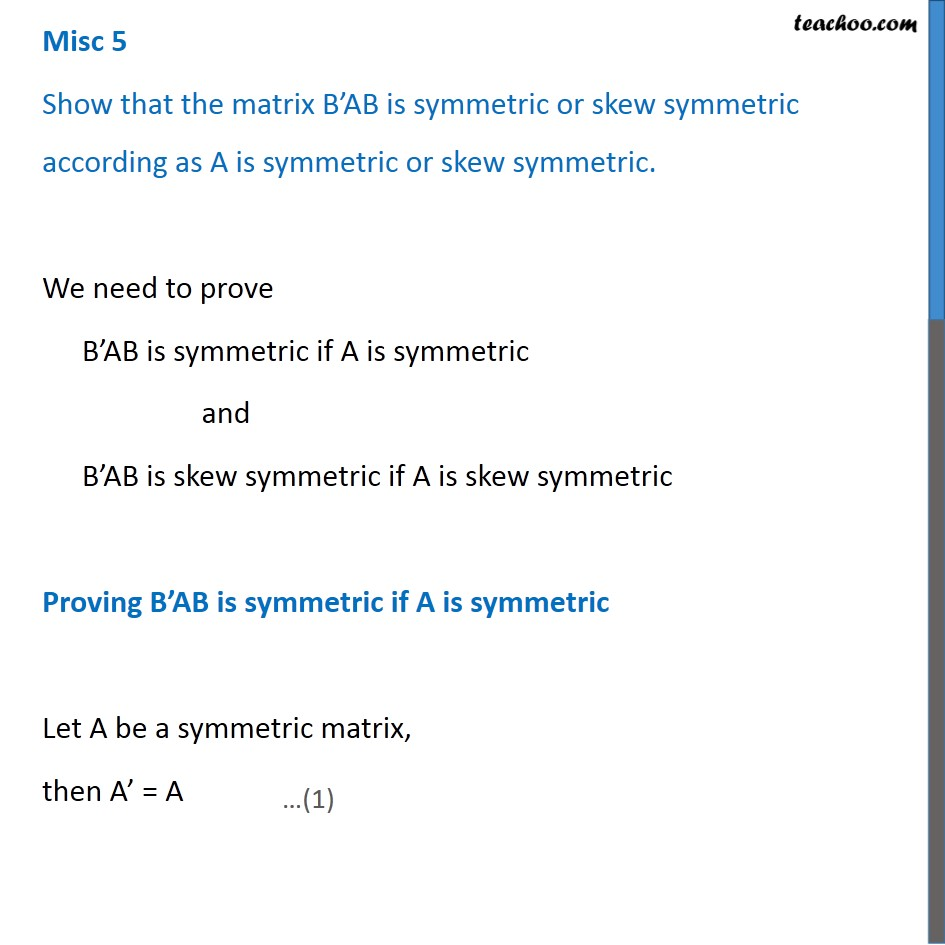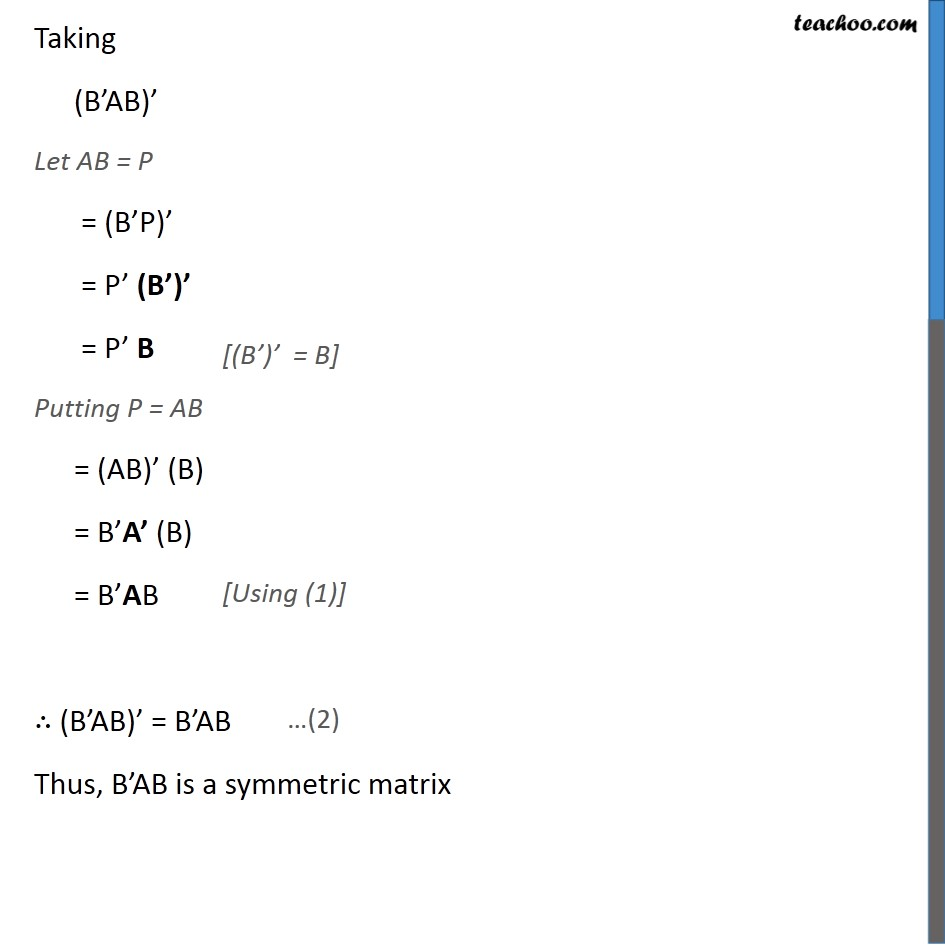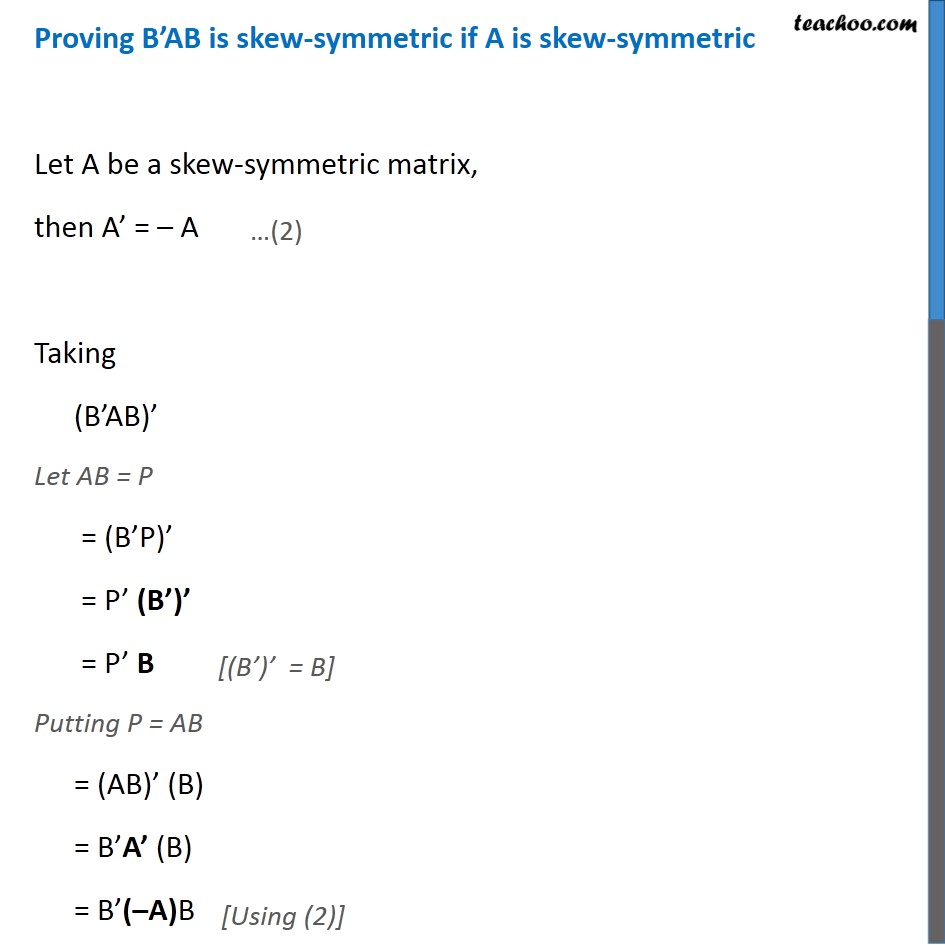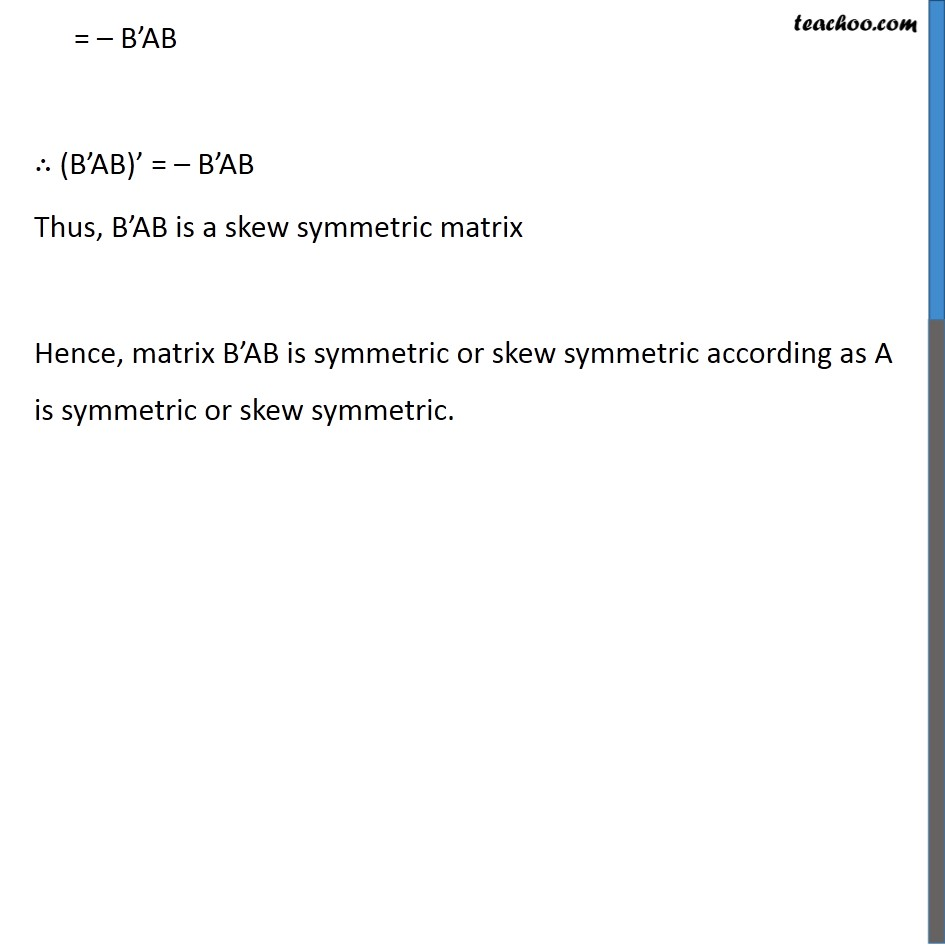1. Chapter 3 Class 12 Matrices
2. Concept wise
3. Proof using property of transpose

Transcript

Misc 5 Show that the matrix B’AB is symmetric or skew symmetric according as A is symmetric or skew symmetric. We need to prove B’AB is symmetric if A is symmetric and B’AB is skew symmetric if A is skew symmetric Proving B’AB is symmetric if A is symmetric Let A be a symmetric matrix, then A’ = A Taking (B’AB)’ Let AB = P = (B’P)’ = P’ (B’)’ = P’ B Putting P = AB = (AB)’ (B) = B’A’ (B) = B’AB ∴ (B’AB)’ = B’AB Thus, B’AB is a symmetric matrix [(B’)’ = B] [Using (1)] Proving B’AB is skew-symmetric if A is skew-symmetric Let A be a skew-symmetric matrix, then A’ = – A Taking (B’AB)’ Let AB = P = (B’P)’ = P’ (B’)’ = P’ B Putting P = AB = (AB)’ (B) = B’A’ (B) = B’(–A)B [(B’)’ = B] = – B’AB ∴ (B’AB)’ = – B’AB Thus, B’AB is a skew symmetric matrix Hence, matrix B’AB is symmetric or skew symmetric according as A is symmetric or skew symmetric.

Proof using property of transpose

About the AuthorDavneet Singh
Davneet Singh is a graduate from Indian Institute of Technology, Kanpur. He has been teaching from the past 10 years. He provides courses for Maths and Science at Teachoo.